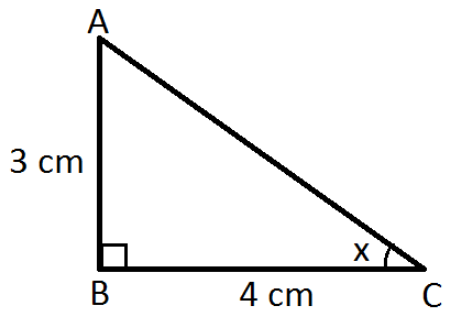# Inverse Tangent Formula

In trigonometry, every function such as sine, cosine and tangent has its inverse function. The tangent formula is used to tan of an angle in a right-angled triangle. The inverse tangent formula is used to find the angle when the side opposite to that angle and adjacent side are known to us. The inverse of Tangent is represented by arctan or tan-1.

The trigonometric functions/ratios are:

• Sine
• Cosine
• Tangent
• Secant
• Cosecant
• Cotangent

The inverse of these trigonometric functions are as follows:

• inverse sine
• inverse cosine
• inverse tangent
• inverse secant
• inverse cosecant
• inverse cotangent

## Tan Inverse Formula

 Tan (A)= Opposite Side / Adjacent  Side A = Tan-1 (Opposite Side/Adjacent Side) where A is an angle

For example, if in a triangle, opposite side to angle A is 1 and the adjacent side is √3

So tan-1 (1/√ 3) = A

As we know, tan 30 = 1/√3

Therefore, tan-1 (tan 30) = A

or A = 30 degrees

## Solved Examples

Example 1: From the given figure, find the value of x.Solution:
From the given,
AB = 3 cm, BC = 4 cm
tan x = AB/BC
tan x = ¾ = 0.75
x = tan-1(0.75)
x = 36.9°

Example 2: If sin x = 0 and cos x = 1, then find the tan-1x.
Solution:
Given,
sin x = 0
x = sin-1(0) = 0
cos x = 1
x = cos-1(1) = 0
Now,
tan-1x = tan-1(0) = 0